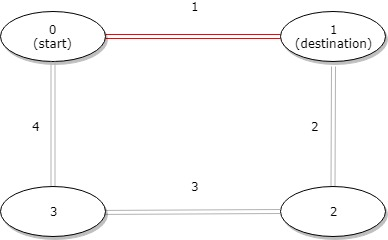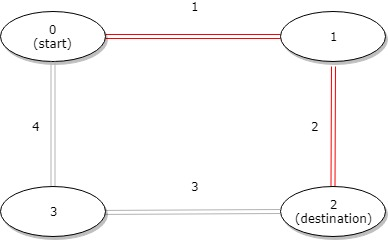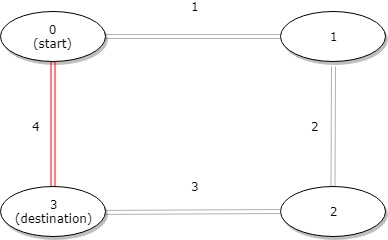# GeetCode Hub

A bus has `n` stops numbered from `0` to `n - 1` that form a circle. We know the distance between all pairs of neighboring stops where `distance[i]` is the distance between the stops number `i` and `(i + 1) % n`.

The bus goes along both directions i.e. clockwise and counterclockwise.

Return the shortest distance between the given `start` and `destination` stops.

Example 1:```Input: distance = [1,2,3,4], start = 0, destination = 1
Output: 1
Explanation: Distance between 0 and 1 is 1 or 9, minimum is 1.```

Example 2:```Input: distance = [1,2,3,4], start = 0, destination = 2
Output: 3
Explanation: Distance between 0 and 2 is 3 or 7, minimum is 3.
```

Example 3:```Input: distance = [1,2,3,4], start = 0, destination = 3
Output: 4
Explanation: Distance between 0 and 3 is 6 or 4, minimum is 4.
```

Constraints:

• `1 <= n <= 10^4`
• `distance.length == n`
• `0 <= start, destination < n`
• `0 <= distance[i] <= 10^4`

class Solution { public int distanceBetweenBusStops(int[] distance, int start, int destination) { } }